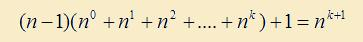# Discovering the Sum of Powers

Back in the days when we were learning binary arithmetic, we came across the following relation between the powers of 2.
If we sum up the lower powers of 2 and add 1 to the result, we get the next power of 2. For instance,
(2^0 + 2^1) + 1 = 2^2
(2^0 + 2^1 + 2^2) + 1 = 2^3 , so on.

Later on, I decided to check if the same relation works for powers of 3. Lets try,
(3^0 + 3^1) + 1 = (1 + 3) + 1 = (4) + 1 = 5.

Nopes, does not work for 3. It falls short of the next power, that is (3^2), by 4. However, we make an important observation. The amount which the result falls short by, is equal to the sum in the bracket (in blue font above), which is 4.
Co-incidence? Let's try another one...

(3^0 + 3^1 + 3^2) + 1 = (1 + 3 + 9) + 1 = (13) + 1 = 14

This falls short of the next power 3^3, by 13. But again, the amount it falls short by is equal to the term in the bracket, i.e. 13. Can't be a co-indicence.

What this means is, to get the desired result, the term in the bracket should appear twice. In other words, the bracket term should be multiplied by 2, which is one less than 3.

Let's check this out again with this correction.

2(3^0 + 3^1) + 1          = 2(1 + 3) + 1       = 8 + 1   = 9.
2(3^0 + 3^1 + 3^2) + 1 = 2(1 + 3 + 9) + 1 = 26 + 1 = 27

It works!

What happens with powers of 4? This time, we will multiply the term in bracket by 3. One less than 4.

3(4^0 + 4^1) + 1          = 3(1 + 4) + 1        = 3(5) + 1   = 15 + 1 = 16.
3(4^0 + 4^1 + 4^2) + 1 = 3(1 + 4 + 16) + 1 = 3(21) + 1 = 63 + 1 = 64.

So we hit upon a general rule. If we sum up the lower powers of number n and add 1, then we have to multiply sum of lower powers by one number less than n, that is n-1, to get the next power of n.

Symbolically,Now, lets prove this property formally. As you may see, proving this is easy enough.

Property :    For natural numbers n >= 1,Proof :
From Algerba, we know that,Applying the formula to n^k+1 and 1^k+1, we get,which means...The number 1 in the bracket can be written as n^0, and hence,Great, we just discovered something new. Now, we know how the sum of smaller powers add up to give higher ones. Having concluded this, I was curious to find out what happens to the reciprocal of the powers? How do they behave?!
Let's find out in the next section - Sum of reciprocal powers

 Quick Comment : * Name Email ( Your email id is safe with us! ) * Comments * Type the number of characters in the word "HUMAN"